# How to use the MOD Function in Excel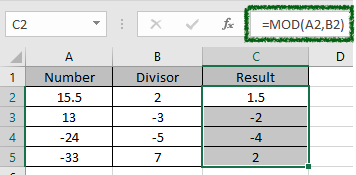In this article, we will learn How to use the MOD function in Excel.

What is MOD or remainder ?

This is a mathematical operator used to calculate the remainder of the division. There are 4 different values involved in a division.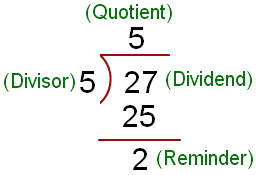So it becomes

Dividend or number = divisor * quotient + remainder

For a division to happen we need Dividend and divisor, all rest are calculated. We can get Quotient value just by using the divide ( / ) operator. But for the remainder value we can use the MOD function in Excel. For example we get quotient 5 by using =27/5 in Excel cells. And calculator the remainder by = 27 - (27/5) * 5 = 2 (remainder). Or you can use =MOD(27,5)

 Operator Division Quotient [ =27/2 ] Remainder [ =MOD(27,2) ] / = 27 / 10 2 7

Let's learn MOD function syntax and an example to illustrate the function usage.

MOD Function in Excel

MOD function is a built-in function to calculate the remainder when the number is divided by the divisor. It always has the same sign as divisor no matter the sign of remainder.

MOD Function Syntax:

 =MOD (number, divisor)

number : dividend or the number to divide

divisor : divisor or the number to divide with

Example :

All of these might be confusing to understand. Let's understand how to use the function using an example. Here Here we have some numbers and their divisors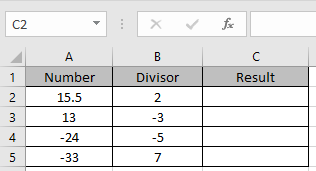Use the formula:

 =MOD(A2,B2)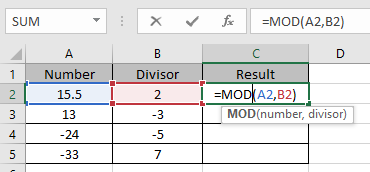Use the formula as shown in the above image. Press Enter to get the result. Then copy and paste this formula in other required cells to apply the same formula on others. Use Ctrl + D or drag down from the right corner of cell till required.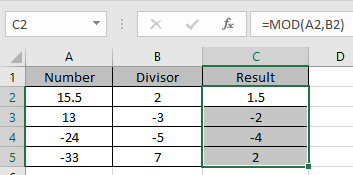As you can see the result for the remainder but the sign ( -ve / +ve) is the same as the sign ( -ve / +ve ) of the divisor.

Here are all the observational notes using the MOD function in Excel
Notes :

1. The formula only works with numbers.
2. The formula returns the number rounded off to any decimal places.
3. Negative argument as decimal rounds the number to integer side.
4. Blank cells as an argument takes as zero value and returns 0 as result, so make sure to check blank cells before.
5. The function returns #VALUE! Error if the text value or numbers as text value.

Hope this article about How to use the MOD function in Excel is explanatory. Find more articles on calculating values and related Excel formulas here. If you liked our blogs, share it with your friends on Facebook. And also you can follow us on Twitter and Facebook. We would love to hear from you, do let us know how we can improve, complement or innovate our work and make it better for you. Write to us at info@exceltip.com.

Related Articles :

How to use the ROUND function in Excel | rounds off the given number to the nearest num_digit decimal using the ROUND function in Excel

How to use the ROUNDUP function in Excel | rounds up the given number to the nearest num_digit decimal using the ROUNDUP function in Excel

How to use the ROUNDDOWN function in Excel | rounds down the given number to the nearest num_digit decimal using the ROUNDDOWN function in Excel

How to use Excel F.TEST Function in Excel : The F.TEST Function is used to calculate F statistic of two samples in excel internally and returns the two tailed probability of the F statistic under Null Hypothesis.

How To Use Excel T.TEST Function in Excel : The T.TEST function is used to determine the confidence of an analysis in Excel. Mathematically, it is used to know if the mean of the two samples are equal or not. T.TEST function is used to accept or reject the null hypothesis.

How to use the DEVSQ Function in Excel : DEVSQ function is a built-in statistical function to calculate the sum of squared deviations from the mean or average of the given distribution provided.

How to calculate Standard Deviation in Excel : To calculate the standard deviation we have different functions in Excel. The standard deviation is the square root of the variance value but It tells more about the dataset than variance.

How to use the VAR function in Excel : Calculate the variance for the sample dataset in excel using the VAR function in Excel.

Popular Articles :

How to use the IF Function in Excel : The IF statement in Excel checks the condition and returns a specific value if the condition is TRUE or returns another specific value if FALSE.

How to use the VLOOKUP Function in Excel : This is one of the most used and popular functions of excel that is used to lookup value from different ranges and sheets.

How to use the SUMIF Function in Excel : This is another dashboard essential function. This helps you sum up values on specific conditions.

How to use the COUNTIF Function in Excel : Count values with conditions using this amazing function. You don't need to filter your data to count specific values. Countif function is essential to prepare your dashboard.

Terms and Conditions of use

The applications/code on this site are distributed as is and without warranties or liability. In no event shall the owner of the copyrights, or the authors of the applications/code be liable for any loss of profit, any problems or any damage resulting from the use or evaluation of the applications/code.